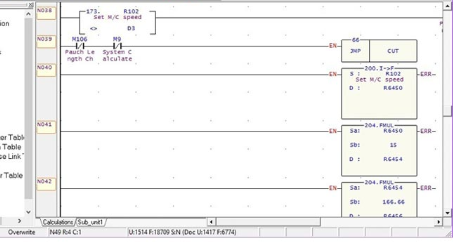# Data Handling Instructions for PLC## Introduction

This session contains general information about the data handling instructions for plc and explains how they function in your application program.

### Convert to BCD (TOD)

Use this instruction to convert 16-bit integers into BCD values. If the integer value you enter is negative, the absolute value of the number is used for conversion.Example

The integer value 9760 stored at N7:3 is converted to BCD and the BCD equivalent is stored in N10:0. The maximum BCD value possible is 9999.Convert from BCD (FRD)

Use this instruction to convert BCD values to integer values.Example

The BCD value 9760 at source N7:3 is converted and stored in N10:0. The maximum source value is 9999, BCD.Use this instruction to convert radians (source) to degrees and store the result in the destination. The following formula applies:

Source * 180/P

where π = 3.141592Use this instruction to convert degrees (source) to radians and store the result in the destination. The following formula applies:

Source * P/ 180

where  π= 3.141592Decode 4 to 1 of 16 (DCD)

When executed, this instruction sets one bit of the destination word.The particular bit that is turned on depends on the value of the first four bits of the source word. See the table below.Encode 1 of 16 to 4 (ENC)

When the rung is true, this output instruction searches the source from the lowest to the highest bit, and looks for the first set bit. The corresponding bit position is written to the destination as an integer as shown in the table below.Copy File (COP) and Fill File (FLL) Instructions

The destination file type determines the number of words that an instruction transfers. For example, if the destination file type is a counter and the source file type is an integer, three integer words are transferred for each element in the counter-type file.Using FLL

This instruction loads elements of a file with either a program constant or value from an element address. The instruction fills the words of a file with a source value. It uses no status bits. If you need an enable bit, program a parallel output that uses a storage address. The following figure shows how file instruction data is manipulated.